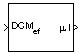# Direction Cosine Matrix ECEF to NED to Latitude and Longitude

Convert direction cosine matrix to geodetic latitude and longitude

• Library:
• Aerospace Blockset / Utilities / Axes Transformations

•## Description

The Direction Cosine Matrix ECEF to NED to Latitude and Longitude block converts a 3-by-3 direction cosine matrix (DCM) into geodetic latitude and longitude. The DCM matrix performs the coordinate transformation of a vector in Earth-centered Earth-fixed (ECEF) axes, (ox0, oy0, oz0), into geodetic latitude and longitude. For more information on the direction cosine matrix, see Algorithms.

## Limitations

The DCM matrix performs the coordinate transformation of a vector in ECEF axes, (ox0, oy0, oz0), into geodetic latitude and longitude. The order of the axis rotations required to bring this about is:

• This implementation generates a geodetic latitude that lies between ±90 degrees, and longitude that lies between ±180 degrees.

• The implementation of the ECEF coordinate system assumes that the origin is at the center of the planet, the x-axis intersects the Greenwich meridian and the equator, the z-axis is the mean spin axis of the planet, positive to the north, and the y-axis completes the right-hand system. For more information, see About Aerospace Coordinate Systems.

## Ports

### Input

expand all

Direction cosine matrix from which to geodetic latitude and longitude, specified as a 3-by-3 matrix.

Data Types: `double`

### Output

expand all

Geodetic latitude and longitude, returned as a 2-by-1 vector in degrees.

Data Types: `double`

## Parameters

expand all

Block behavior when direction cosine matrix is invalid (not orthogonal).

• `Warning` — Displays warning indicating that the direction cosine matrix is invalid.

• `Error` — Displays error indicating that the direction cosine matrix is invalid.

• `None` — Does not display warning or error (default).

#### Programmatic Use

 Block Parameter: `action` Type: character vector Values: `'None'` | `'Warning'` | `'Error'` Default: `'None'`

Data Types: `char` | `string`

Tolerance of the direction cosine matrix validity, specified as a scalar. The block considers the direction cosine matrix valid if these conditions are true:

• The transpose of the direction cosine matrix times itself equals `1` within the specified tolerance (`transpose(n)*n == 1±tolerance`).

• The determinant of the direction cosine matrix equals `1` within the specified tolerance (`det(n) == 1±tolerance`).

#### Programmatic Use

 Block Parameter: `tolerance` Type: character vector Values: `'eps(2)'` | scalar Default: `'eps(2)'`

Data Types: `double`

## Algorithms

The DCM matrix performs the coordinate transformation of a vector in ECEF axes, (ox0, oy0, oz0), into geodetic latitude and longitude. The order of the axis rotations required to bring this about is:

1. A rotation about oz0 through the longitude (ι) to axes (ox1, oy1, oz1)

2. A rotation about oy1 through the geodetic latitude (μ) to axes (ox2, oy2, oz2)

`$\begin{array}{l}\left[\begin{array}{c}o{x}_{2}\\ o{y}_{2}\\ o{z}_{2}\end{array}\right]=DC{M}_{ef}\left[\begin{array}{c}o{x}_{0}\\ o{y}_{0}\\ o{z}_{0}\end{array}\right]\\ \\ \left[\begin{array}{c}o{x}_{2}\\ o{y}_{2}\\ o{z}_{2}\end{array}\right]=\left[\begin{array}{ccc}-\mathrm{sin}\mu & 0& \mathrm{cos}\mu \\ 0& 1& 0\\ -\mathrm{cos}\mu & 0& -\mathrm{sin}\mu \end{array}\right]\left[\begin{array}{ccc}\mathrm{cos}\iota & \mathrm{sin}\iota & 0\\ -\mathrm{sin}\iota & \mathrm{cos}\iota & 0\\ 0& 0& 1\end{array}\right]\left[\begin{array}{c}o{x}_{0}\\ o{y}_{0}\\ o{z}_{0}\end{array}\right]\end{array}$`

Combining the two axis transformation matrices defines the following DCM.

`$DC{M}_{ef}=\left[\begin{array}{ccc}-\mathrm{sin}\mu \mathrm{cos}\iota & -\mathrm{sin}\mu \mathrm{sin}\iota & \mathrm{cos}\mu \\ -\mathrm{sin}\iota & \mathrm{cos}\iota & 0\\ -\mathrm{cos}\mu \mathrm{cos}\iota & -\mathrm{cos}\mu \mathrm{sin}\iota & -\mathrm{sin}\mu \end{array}\right]$`

To determine geodetic latitude and longitude from the DCM, the following equations are used:

`$\begin{array}{l}\mu =\text{asin}\left(-DCM\left(3,3\right)\right)\\ \\ \iota =\text{atan}\left(\frac{-DCM\left(2,1\right)}{DCM\left(2,2\right)}\right)\end{array}$`

 Zipfel, Peter H., Modeling and Simulation of Aerospace Vehicle Dynamics. Second Edition. Reston, VA: AIAA Education Series, 2000.

 Recommended Practice for Atmospheric and Space Flight Vehicle Coordinate Systems, R-004-1992, ANSI/AIAA, February 1992.

 Stevens, B. L., and F. L. Lewis. Aircraft Control and Simulation, Hoboken, NJ: John Wiley & Sons, 1992.

## SupportGet trial now